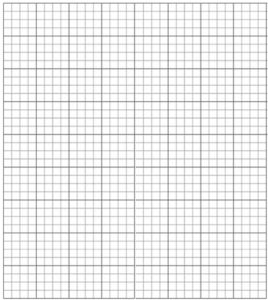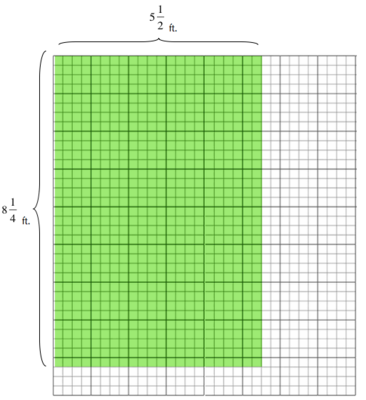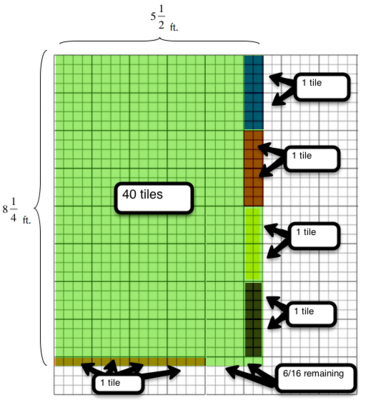# Chavone's Bathroom Tiles

Alignments to Content Standards: 5.NF.B.4.b

Chavone is remodeling her bathroom.  She plans to cover the bathroom floor with tiles that are each 1 square foot.  Her bathroom is $5\frac12$ feet wide and $8\frac14$  feet long.

How many tiles will she need to cover the floor? Give an exact answer that includes the fractions of a tile she will need. Each unit square has been broken into sixteenths to allow precise measurements. Use the figure below to illustrate your answer.## IM Commentary

This task helps students link the concepts of multiplication and area.  Students first learn how these concepts reinforce each other with whole numbers in third grade.  In fifth grade, they extend this understanding beyond whole numbers to fractions and decimals.

Whether students are multiplying multi-digit whole numbers or mixed numbers, they often forget to consider all of the partial products.  When students begin problems like 14 x 82, they often jump to claim that the product will be 808 because 10 times 80 is 800 and 4 x 2 is 8, therefor the product is 808. Exposing students to the area model is crucial in helping students understand why this product is not 808.  Likewise, in this task it is tempting to assume that multiplying 5 and 1/2 and 8 and 1/4 would yield a product of 40 and 1/8 through the same reasoning above.  It may be helpful to have students make a prediction about how many tiles Chavone will need-- a student will likely state that she will need 40 and 1/8 tiles.  After having students explore the area model, this misconception should be explicitly addressed since it will come up often as fifth graders begin multiplying mixed numbers.

Lastly, teachers should carefully address the role of area in this problem.  This task is asking us to find the number of tiles, but we must do that by finding the total number of square feet Chavone will need to cover.  The concept of tiling is helpful to students in understanding area as the number of square units needed to cover a plane without gaps or overlaps.  The concept of tiling is useful as student first explore area with rational numbers.  When students have more experience with finding the area contained by rectangles with rational side lengths, they may transition to more abstract representations such as the amount of carpeting needed to cover a floor or the amount of soil needed to cover a garden bed.

## Solution

The grid below shows the area of Chavone's bathroom floor that she needs to cover with tiles.If we break the area up into whole tiles and parts of tiles needed it would look like this:This area model shows us that Chavone would need 45 and 6/16 (or 45 and 3/8) tiles to exactly cover her bathroom floor.

We can also see that, as with whole numbers, when we multiply the side-lengths of the rectangle, we can find the area:

\begin{align} 8\frac14 \times 5 \frac12 &= \frac{33}{4}\times\frac{11}{2} \\ &= \frac{363}{8} \\ &= 45\frac38 \end{align}

The fact that this always works not just for whole numbers, but also for rational numbers, is very deep, and this fact warrants its own investigation.